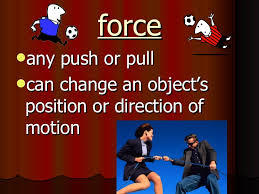## 7th GRADE SCIENCE : Article 20 : Ending Forces lesson 1. Final and review.* Activity 1.34   :   Force lesson 1.     Reviews.                                       ( 10/23/17   Monday )

1.34.a   Pics of earth, gravity and weight to make the poster.         ( 10 points ).

1.34.b   On the poster you must write a weight and gravitys description.   ( 5 points ).

1.34.c   Fun way to review forces.              ( 5 points ).

Copy this outline on a sheet in order to review some of the forces studied.

* Activity 1.35   :   Listing the main forces studied at the LESSON 1.         ( 10/24/17   Tuesday )

FINAL REVIEW  LESSON 1 :
FORCES AND MOTION.

- These are the main concepts you have studied in this unit :

( What a force is, forces effects, vectors, kind of forces, tension force, spring force, air resistance force, nuclear force, gravitational force, friction force, normal force, electrical force, magnetic force, applied force, contact forces, non-contact forces, weight force, gravity force, electromagnet, earths inner forces, tectonic plates, elastic force, dynamometer, escalar and vectorial magnitudes, application point, speed, velocity, direction, opposite direction, units, newtons, Isaac Newton, Robert Hooke, acceleration, Newtons laws, Hookes law, motion, inertia, F=K.X , F= m.a , balance force, stiffen structures, calculations, net force, reaction force, etc ).

1.35.a   Look up the following links and do a list in google docs with the forces studied in this first lesson .  As well as naming them, explain something about 15 of them describing what they are and which effects they can cause.

-  When you finish email it to me.

( 20  points ).

LINK 1   ( Kind of forces ).

LINK 2  ( Weight , mass , gravity ).

LINK 3  ( Tectonic plates ).

LINK 4  ( Magnitude and vectors , direction ).

LINK 6    ( Elastic force , Hookes law ).

LINK 7  ( Newtons laws, acceleration , motion , inertia ).

LINK 8  ( Net force game ).

LINK 9  ( Net force ).

LINK 10  ( Stiffening structures ).

LINK 11  ( Earth's layers ).

Comentarios  Ir a formulario